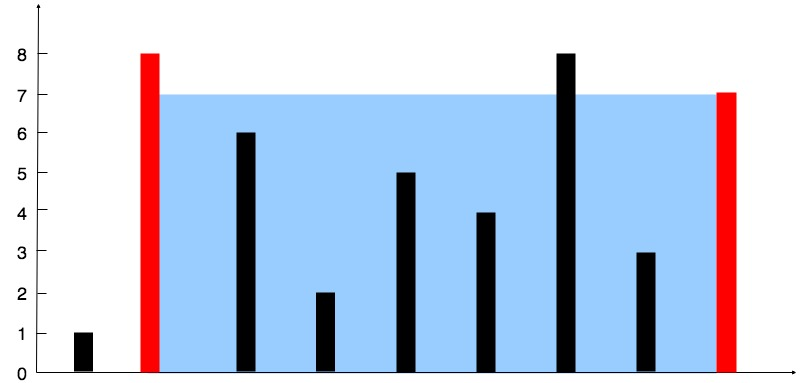# 1. 首尾双指针

## 167 两数之和II-输入有序数组

输入: numbers = [2, 7, 11, 15], target = 9



vector<int> twoSum(vector<int>& numbers, int target) {
int l =0,r=numbers.size()-1;
if(numbers.size()==0) return vector<int>{-1,-1};
while(l<r)
{
if(numbers[l]+numbers[r]==target)
return vector<int>{l+1,r+1};
else if(numbers[l]+numbers[r]<target)
l++;
else
r--;
}
return vector<int>{-1,-1};
}


## 015 三数之和

例如, 给定数组 nums = [-1, 0, 1, 2, -1, -4]，

[
[-1, 0, 1],
[-1, -1, 2]
]


vector<vector<int>> threeSum(vector<int>& nums) {
set<vector<int>> s;
vector<vector<int>> res;
sort(nums.begin(),nums.end());
if (nums.size() < 2) return res;
for(int i=0;i<nums.size()-1;i++)
{
int l=i+1,r=nums.size()-1;
while(l<r)
{
if(nums[i]+nums[l]+nums[r]==0)
{
vector<int> tmp={nums[i],nums[l],nums[r]};
s.insert(tmp);
l++;r--;
}
else if(nums[i]+nums[l]+nums[r]<0) l++;
else  r--;
}
}
for(auto x:s)
res.push_back(x);
return res;
}


• 注意push进入结果时，l和r也需要增加或减少，不然会超时。

• lr去重时要注意，l++r--用两次

• for去重时，应该要加入i>0的判断，不然会溢出。
• 应该nums[l]==nums[l+1]而不是nums[l-1]==nums[l]这样会和i重合
vector<vector<int>> threeSum(vector<int>& nums) {
sort(nums.begin(), nums.end());
vector<vector<int>> res;
if (nums.size() < 2) return res;
for (int i = 0; i < nums.size() - 2; i++)
{
//对i去重
if (i > 0 && nums[i] == nums[i - 1])
continue;
int l = i + 1, r = nums.size() - 1;
int sum = 0 - nums[i];
while (l < r)
{
if (nums[l] + nums[r] == sum)
{
res.push_back(vector<int>{nums[i], nums[l], nums[r]});
while (l < r && nums[l] == nums[l + 1]) l++;
while (l < r && nums[r] == nums[r - 1]) r--;
l++;
r--;
}
else if (nums[l] + nums[r] < sum)
{
while (l < r && nums[l] == nums[l + 1]) l++;
l++;
}
else
{
while (l < r && nums[r] == nums[r - 1]) r--;
r--;
}
}
}
return res;
}


## 016 最接近的三数之和

例如，给定数组 nums = [-1，2，1，-4], 和 target = 1.



int threeSumClosest(vector<int>& nums, int target) {
if (nums.size() < 3) return 0;
sort(nums.begin(),nums.end());
int closet = accumulate(nums.begin(),nums.begin()+3,0);
for(int i=0;i<nums.size()-2;i++)
{
int l=i+1,r=nums.size()-1;
while(l<r)
{
int sum=nums[l]+nums[r]+nums[i];
if(sum==target) return sum;
if(abs(sum-target)<abs(closet-target))
closet=sum;
else if(sum<target) l++;
else r--;
}
}
return closet;
}


## 018 四数之和

给定数组 nums = [1, 0, -1, 0, -2, 2]，和 target = 0。

[
[-1,  0, 0, 1],
[-2, -1, 1, 2],
[-2,  0, 0, 2]
]


vector<vector<int>> fourSum(vector<int>& nums, int target) {
vector<vector<int>> res;
if(nums.size()<4) return res;
sort(nums.begin(),nums.end());
for(int i=0;i<nums.size()-3;i++)
{
if(i!=0&&nums[i]==nums[i-1]) continue;
for(int j=i+1;j<nums.size()-2;j++)
{
if(j!=i+1&&nums[j]==nums[j-1]) continue;
int l=j+1,r=nums.size()-1;
int sum12=nums[i]+nums[j];
while(l<r)
{
int sum34=nums[l]+nums[r];
if(sum12+sum34==target)
{
vector<int> tmp{nums[i],nums[j],nums[l],nums[r]};
res.push_back(tmp);
while(l<r&&nums[l]==nums[l+1]) l++;
while(l<r&&nums[r]==nums[r-1]) r--;
l++;
r--;
}
else if(sum12+sum34<target)
{
while(l<r&&nums[l]==nums[l+1]) l++;
l++;
}
else
{
while (l < r && nums[r] == nums[r - 1]) r--;
r--;
}
}
}
}
return res;
}


## 011 乘最多水的容器int maxArea(vector<int>& height) {
int l=0,r=height.size()-1;
int maxarea = 0;
while(l<r)
{
int area = min(height[l],height[r])*(r-l);
maxarea=max(maxarea,area);
if(height[l]<height[r]) l++;
else r--;
}
return maxarea;
}


## 075 颜色分类

输入: [2,0,2,1,1,0]



void sortColors(vector<int>& nums) {
int zerotail = 0, twohead = nums.size() - 1;
while (zerotail < nums.size() && nums[zerotail] == 0) zerotail++;
int index = zerotail;
{
if (nums[index] == 0)
std::swap(nums[zerotail++], nums[index++]);
else if (nums[index] == 2)
else
index++;
}
}


# 2. 同向双指针

## 003 无重复字符的最长子串

输入: "abcabcbb"



int lengthOfLongestSubstring(string s) {
set<char> cache;
int l=0,r=0;
int maxlen=0;
while(r<s.size())
{
if(cache.find(s[r])==cache.end())
{
cache.insert(s[r++]);
maxlen=max(maxlen,r-l);
}
else
cache.erase(s[l++]);
}
return maxlen;
}


## 209 长度最小的子数组

输入: s = 7, nums = [2,3,1,2,4,3]



int minSubArrayLen(int s, vector<int>& nums) {
if (nums.empty())
return 0;
int left = 0, right = 0, sum = 0, len = nums.size();
int res = INT_MAX;

while (right < len)
{
while (sum < s && right < len)
sum += nums[right++];
while (sum >= s)
{
res = min(res, right - left);
sum -= nums[left++];
}
}
return res == INT_MAX ? 0 : res;
}


# 3. 其他双指针

## 028 实现strStr()

输入: haystack = "hello", needle = "ll"



int strStr(string haystack, string needle) {
if (needle == "") return 0;
int i = 0;
for (; i < haystack.size(); i++)
{
if (haystack[i] != needle) continue;
int j = 0;
for (; j < needle.size(); j++)
{
if (haystack[i + j] != needle[j])
break;
}
if (j == needle.size()) return i;
}
return -1;
}


## 088 合并两个有序数组

输入:
nums1 = [1,2,3,0,0,0], m = 3
nums2 = [2,5,6],       n = 3



void merge(vector<int>& nums1, int m, vector<int>& nums2, int n) {
int i=m-1,j=n-1;
int len=nums1.size()-1;
while(i>=0&&j>=0)
{
if(nums1[i]>nums2[j])
nums1[len--]=nums1[i--];
else
nums1[len--]=nums2[j--];
}
while(j>=0) nums1[len--]=nums2[j--];
}